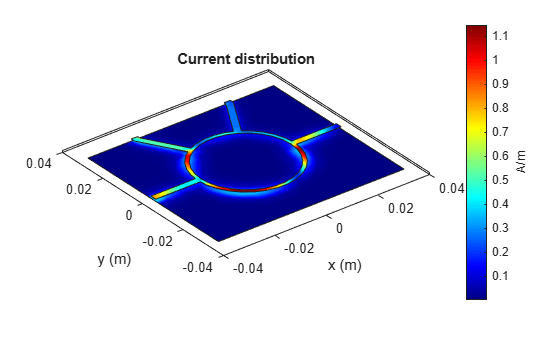# Introduction to 4-Port Couplers

This example shows how to design and analyze 4-Port Couplers.

4-Port couplers have a through port, coupled port, and an isolated port. For the equal split couplers, power is evenly split between the through port and the coupled port and the ideal coupling factor is 3 dB. No power is coupled to the isolated port. In this example, you will design and analyze a Branchline coupler and Ratrace coupler.

### Design and Analyze Branchline Coupler

The Branchline coupler is a 3-dB coupler and divides power equally between the through and coupled arms. It is also called Quadrature Hybrid because of the 90 degree phase difference in the outputs from the through and coupler arms. With all ports matched, power entering port 1 is evenly divided between ports 2 and 4, with a 90 degree phase shift between the outputs. No power is coupled to the Port 3 (the isolated port).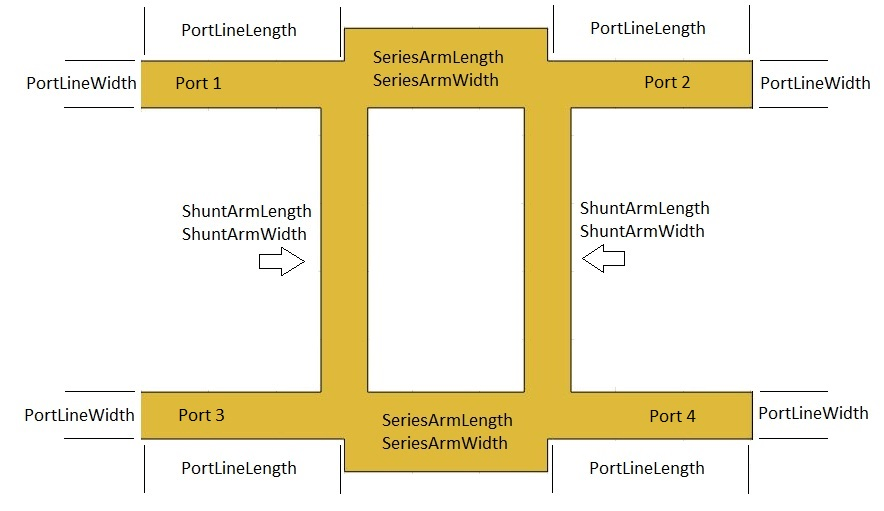Use the object couplerBranchline to create a Branchline coupler and visualize it.

```coupler = couplerBranchline; figure; show(coupler);```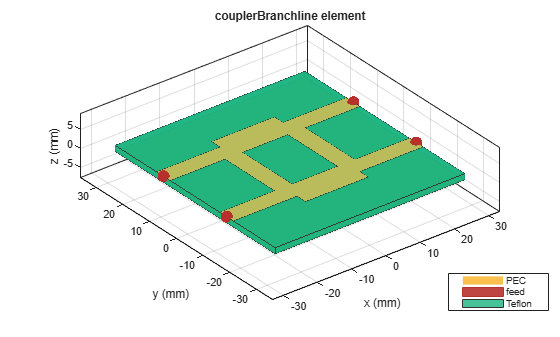The `Height` property controls the substrate thickness for the RF PCB Catalog elements. Changing the Height property changes the substrate thickness.

Change the `Height` property of the Branchline coupler to 0.508 mm . Use the `design `function to design the Branchline coupler at 3 GHz and visualize it.

```coupler.Height = 0.508e-3; coupler = design(coupler,3e9); figure; show(coupler);```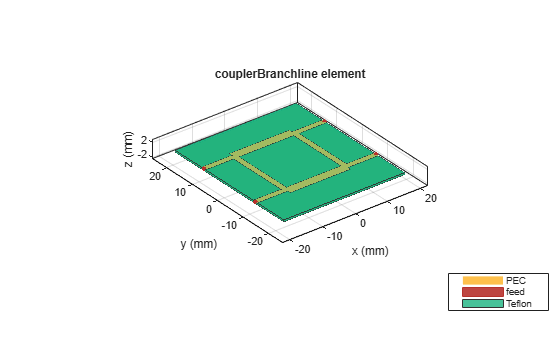Use the `sparameters` function to calculate the S-Parameters and plot it using the `rfplot` function.

```spar = sparameters(coupler,linspace(1e9,5e9,31)); figure rfplot(spar)```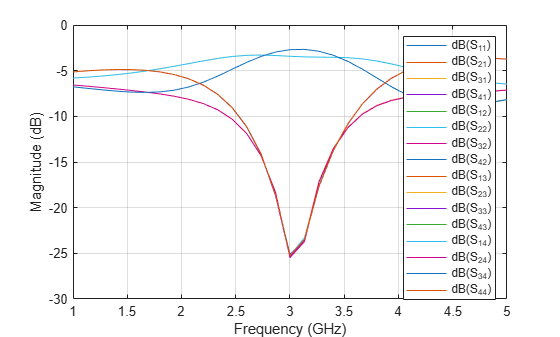As there are 16 curves in the result, let us analyze the result in three different parts.

### Plot Return Loss Values at All Ports

```figure rfplot(spar,'diag')```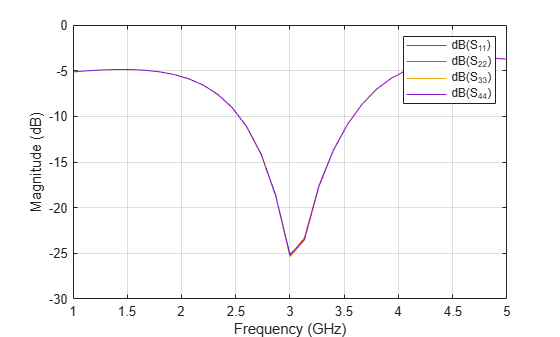The result shows that all the ports are a good match at 3 GHz and the value of S11, S22, S33, and S44 is close to -30 dB.

### Plot S-Parameters When Input is Applied at Port 1

Analyze the values of S21, S31, and S41 to understand the behavior of Branchline coupler when input is applied to port 1.

```figure rfplot(spar,2:4,1)```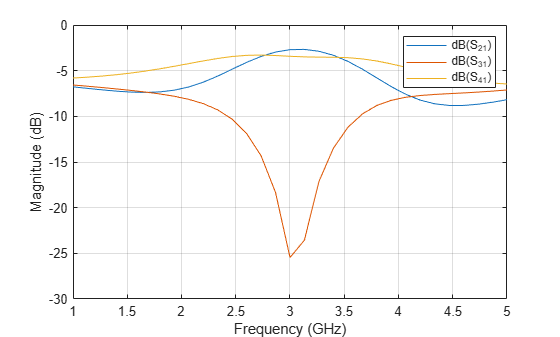The result shows that the power is equally divided between Port 2 and Port 4. Port 3 is isolated.

Plot the phase of the S21 and S41 to understand the phase different between the output ports.

`figure, rfplot(spar,[2 4],1,'angle')`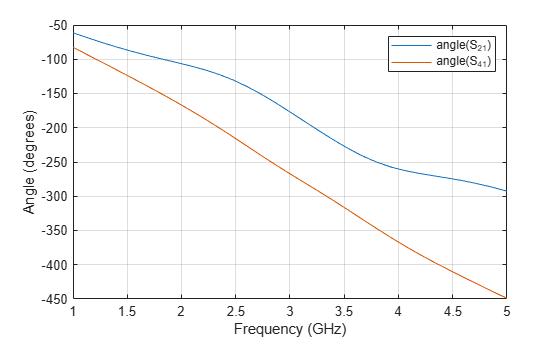The result shows that the phase difference between the output ports is close to 90 degrees at the design frequency. Hence, the outputs at Port 2 and Port 4 is out of phase by 90 degrees when the input is applied to Port 1.

### Plot S-Parameters When Input is Applied at Port 3

Analyze the values of S13, S23, and S43 to understand the behavior of Branchline coupler when input is applied to Port 3.

```figure rfplot(spar,[2 4 1],3)```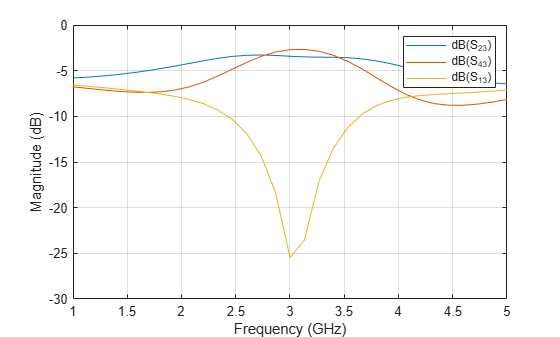The result shows that the power is equally divided to Port 2 and Port 4. Port 1 is isolated.

Plot the phase of the S23 and S43 to understand the phase different between the output ports.

```figure rfplot(spar,[2 4],3,'angle')```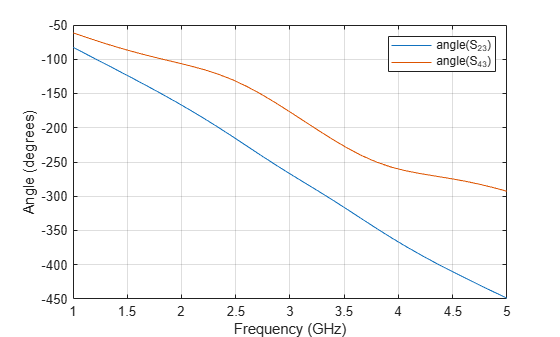The result shows that the phase difference between the output ports is close to 90 degrees at the design frequency of 3 GHz. Hence, when the input is applied to Port 3, the output at Port 2 and Port 4 is out of phase by 90 degree.

Use the `current` method to visualize the current distribution on the Branchline coupler

```figure current(coupler,3e9)```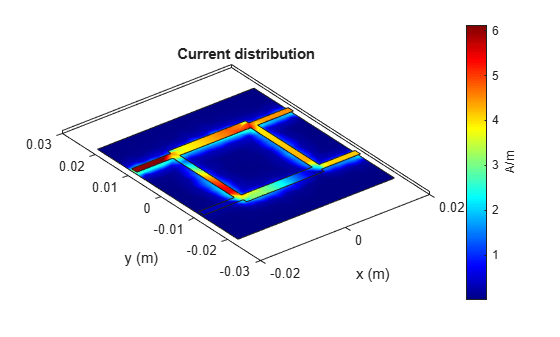### Design and Analyze Ratrace Coupler

The Ratrace Coupler is a 4-port coupler and is also called a 180 deg ring hybrid as the output ports exhibit a phase shift of 180 deg. With reference to the 180 deg hybrid shown below, a signal applied at Port 3 will be evenly split into two in-phase components at Ports 2 and 4. Port 1 is isolated. If the input is applied at Port 1, signal is equally split into two components with a 180 deg phase difference at Ports 2 and 4. Port 3 is isolated. When operated as a combiner, with input signals applied at Ports 2 and 4, the sum of the inputs forms at Port 3, while the difference forms at Port 1. Hence Port 3 and 1 are referred to as the sum and difference ports respectively.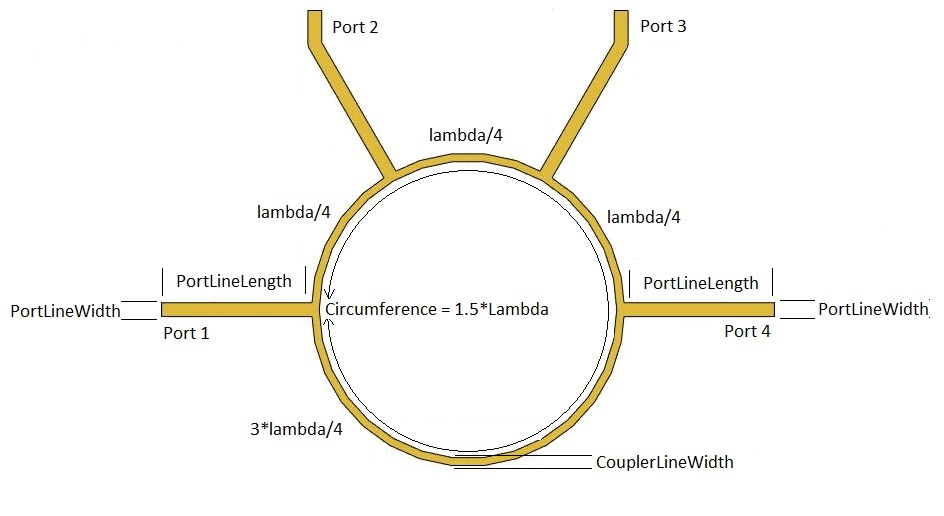Use the couplerRatrace object to create a Ratrace coupler and visualize it.

```coupler = couplerRatrace; figure show(coupler)```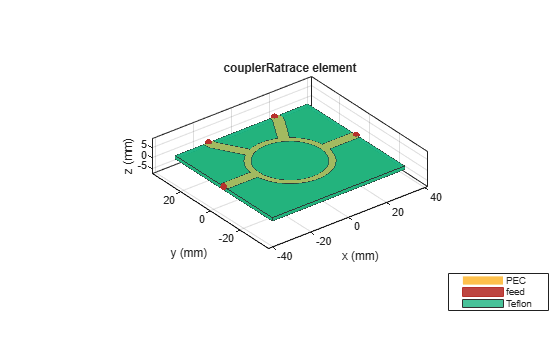Change the Height property for the Ratrace coupler. Use the design function to design the Ratrace coupler at 3 GHz and visualize it.

```coupler.Height = 0.762e-3; coupler = design(coupler,3e9); figure show(coupler)```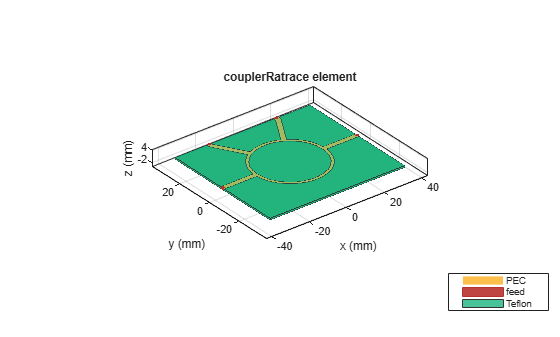Use the `sparameters` function to calculate the S-Parameters for the Ratrace coupler and plot it using the `rfplot` function.

```spar = sparameters(coupler,linspace(1e9,5e9,31)); figure rfplot(spar)```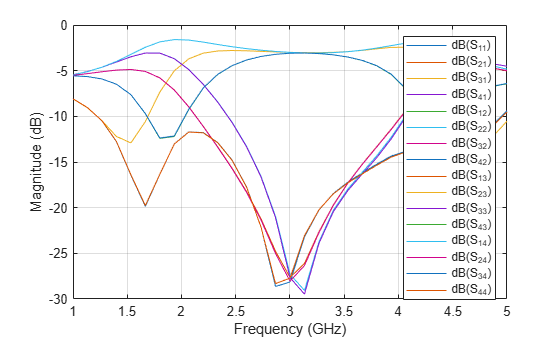As there are 16 curves in the result, let us analyze the result in three different parts.

### Plot Return Loss Values at All Ports

```figure rfplot(spar,'diag')```The result shows that all the ports are a good match at 3 GHz and the values of S11, S22, S33, and S44 is higher than -30 dB.

### Plot S-Parameters When Input is Applied at Port 1

Analyze the values of S21, S31, and S41 to understand the behavior of Ratrace coupler when input is applied to port 1.

```figure rfplot(spar,2:4,1)```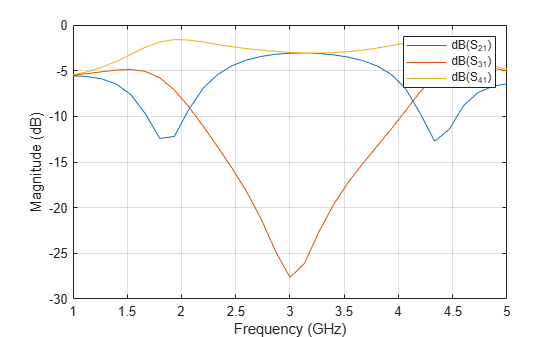The result shows that the power is equally divided between Port 2 and Port 4. Port 3 is isolated when the input is applied to Port 1.

Plot the phase of S21 and S41 to understand the phase difference between the output ports.

```figure rfplot(spar,[2 4],1,'angle')```The result shows that the phase difference between the output ports is close to 180 degrees at the design frequency. Hence, when the input is applied at Port 1, the output at Port 2 and Port 4 is out of phase by 180 degrees.

### Plot S-Parameters When Input is Applied at Port 3

Analyze the values of S23, S43, and S13 to understand the behavior of Ratrace coupler when input is applied to Port 3.

```figure rfplot(spar,[2 4 1],3)```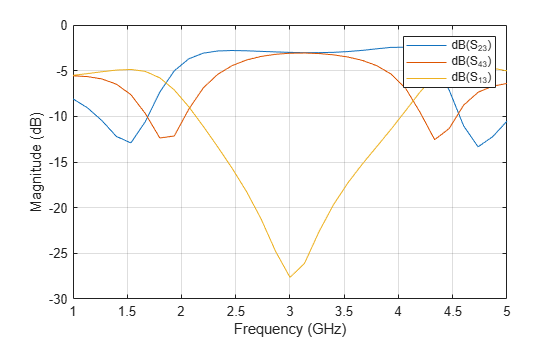The result shows that the power is equally divided between Port 2 and Port 4. Port 1 is isolated when the input is applied to Port 3.

Plot the phase of the S23 and S43 to understand the phase difference between the output ports.

```figure rfplot(spar,[2 4],3,'angle')```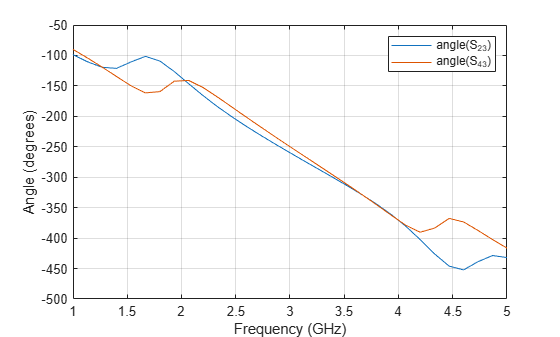The result shows that the phase difference between the output ports is close to 0 degrees at the design frequency of 3 GHz. Hence, when the input is applied at Port 3, the output at Port 2 and Port 4 is in phase.

Use the `current` method to visualize the current distribution on the Ratrace coupler

```figure current(coupler,3e9)```